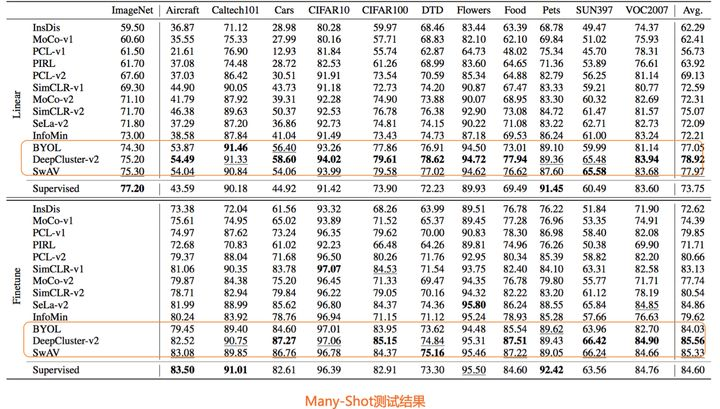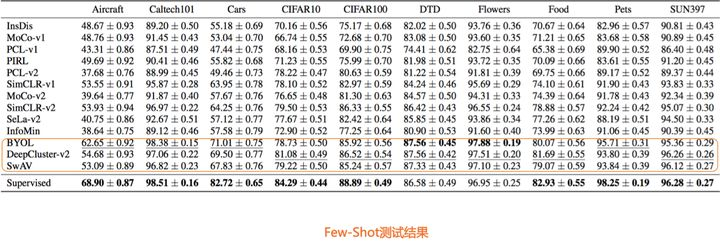“但是，既然NLP这样做（自监督，无需标注数据）成功了，图像领域难道就不能成功吗？”我相信，追寻这个问题的答案，应该是促使很多人，从图像领域的有监督预训练，向自监督预训练转向的重要心理支撑。目前看，虽然说不太容易，但也算曙光乍现，而这道曙光，正是对比学习。

### 基于负例的对比学习：以SimCLR为例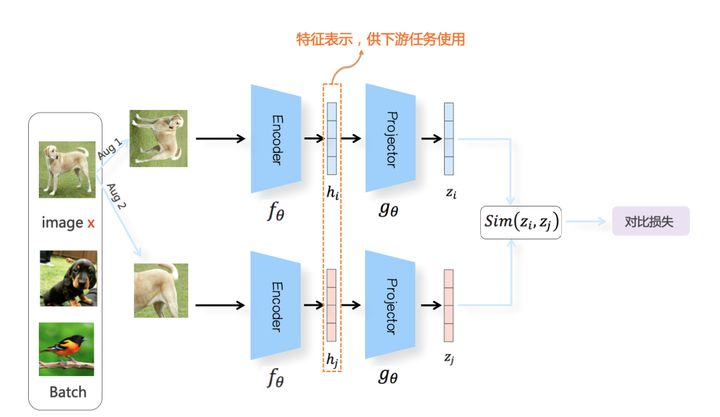$S(x_i,x_j) = \frac{z_i^Tz_j}{\Vert z_i \Vert_2 \Vert z_j \Vert_2}$

$L_i = -log (\frac{exp(S(z_i,z_i^+) / \tau)}{\sum_{j=0}^K exp(S(z_i,z_j)/ \tau)})$

SimCLR论文中，对于Projector和Encoder的编码差异进行了对比实验，结论是：Encoder后的特征表示，会有更多包含图像增强信息在内的细节特征，而这些细节信息经过Projector后，很多被过滤掉了。虽然为何需要两次非线性变换，目前只有实验结果，并未有理论解释。我个人猜测，可能是如下原因：我们知道，一般的特征抽取器，在做特征提取的时候，底层偏向抽取通用的低层特征，往往与任务无关，通用性强；接近比如分类任务的高层网络结构，更倾向编码任务相关的高阶特征信息。想来，Encoder和Projector也应该如此，也就是说，在接近任务的高层网络，也就是Projector，会编码更多跟对比学习任务相关的信息，低层就是Encoder，会编码更多跟任务无关的通用细节信息。对于下游任务，这种对比学习训练任务相关的特征，可能会带来负面影响。如果映射网络只包含Encoder的话，那么特征表示里会有很多预训练任务相关特征，会影响下游任务效果；而加上Projector，等于增加了网络层深，这些任务相关特征就聚集在Projector，此时Encoder则不再包含预训练任务相关特征，只包含更通用的细节特征。这是为何需要两次映射过程，我猜大致是这个原因，但也纯属无依据猜测，不保证正确性。

### 秘境召唤：对比学习到底在干什么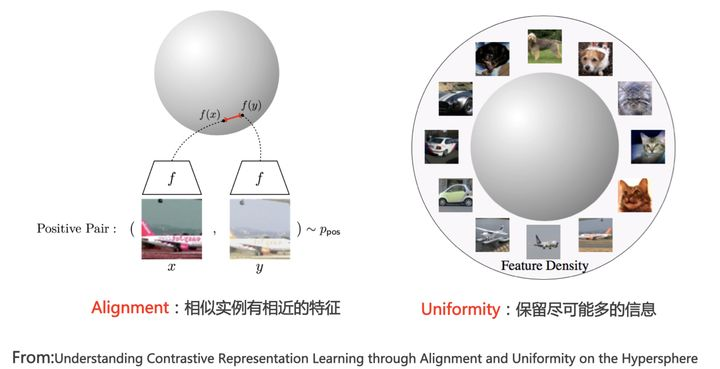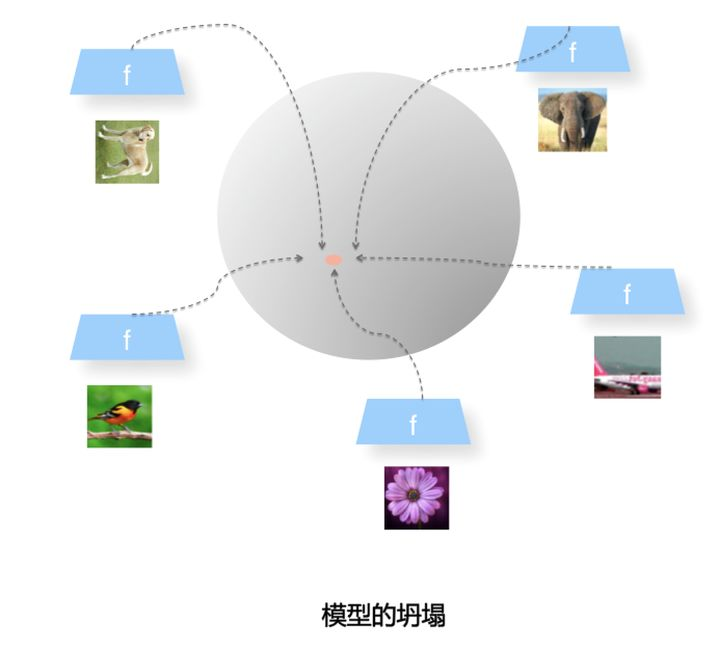Uniformity特性的极端反例，是所有数据映射到单位超球面同一个点上，这极度违背了Uniformity原则，因为这代表所有数据的信息都被丢掉了，体现为数据极度不均匀得分布到了超球面同一个点上。也就是说，所有数据经过特征表示映射过程 $g_{\theta}(f_{\theta}(x))$ 后，都收敛到了同一个常数解，一般将这种异常情况称为模型坍塌（Collapse）。如果对比学习的损失函数定义不好，非常容易出现模型坍塌的情形（参考上图）。

$L_i = -log (\frac{exp(S(z_i,z_i^+) / \tau)}{\sum_{j=0}^K exp(S(z_i,z_j)/ \tau)})$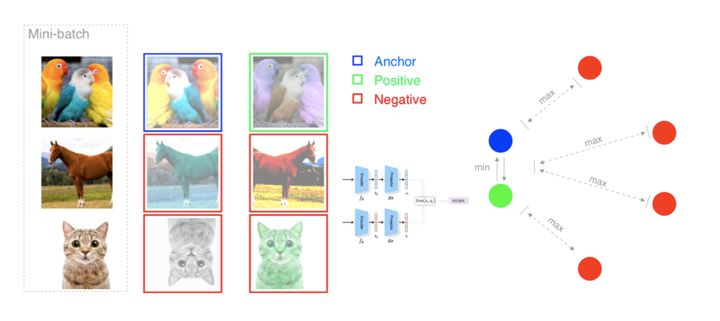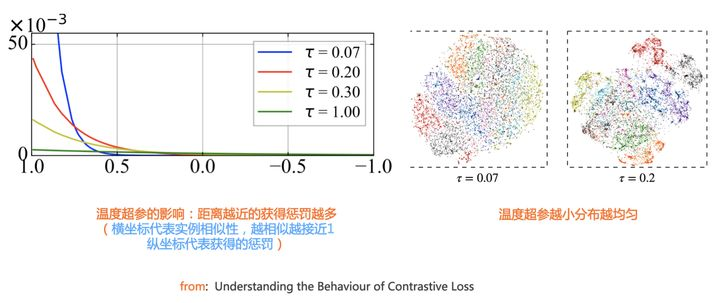### 基于负例的对比学习：Batch之外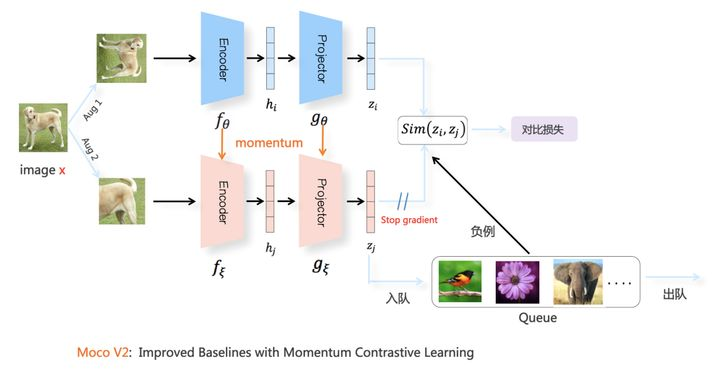Moco V2的模型结构如上图所示。其图像增强方法、Encoder结构、Projector结构、相似性计算方法以及InfoNCE损失函数，和SimCLR基本一致。最主要的特点和创新在两个分枝中的下分枝：SimCLR里上下两个分枝是对称的，两者可参数共享，而Moco V2的下分枝模型参数更新，则采用了动量更新（Momentum Update）机制。下面我们简单介绍下这种动量更新机制，这个机制目前已经在很多其它领域被广泛采用。

Moco V2的下分枝，也由序列的Encoder和Projector两次非线性映射构成，两个构件的模型结构和上分枝对应结构保持一致，但是自身独有一套参数。这套模型参数的更新，并不是通过常规的损失函数反向传播来进行梯度更新，而是采用如下的移动平均（Moving Average）机制进行更新：

$\xi = m\xi +(1-m)\vartheta$

Moco V2维护了一个较大的负例队列，当对比学习需要在正例和负例之间进行对比计算时，就从这个负例队列里取K个，K数值可以根据需要调整大小，但是已经不局限于Batch Size的限制了。下分枝的动量更新模型结构有两个作用：一个是将第二组图像增强视图Aug2里的图像，映射到对应的表示空间编码 $z_j$ ，为第一组图像增强视图Aug1提供正例；第二个作用是更新负例队列里数据的图像表示编码：一般会将最新Batch里Aug2对应的特征表示编码放入队列，而最老的那个Batch对应的图像编码出队，这样就可以不断更新负例队列内负例编码内容。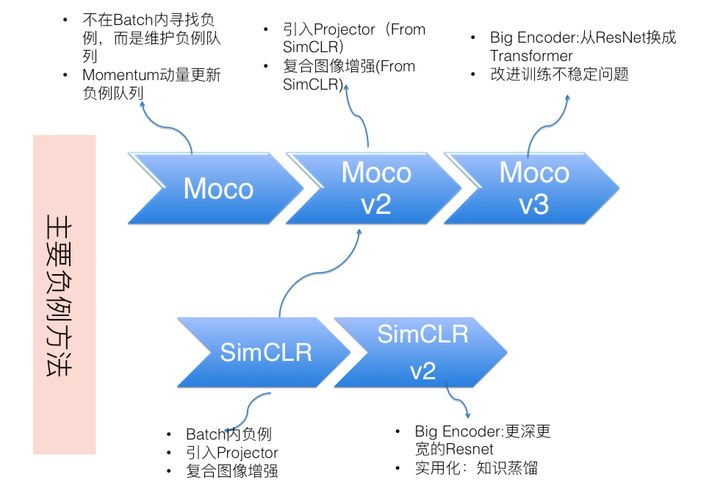### 对比聚类：负例隐身术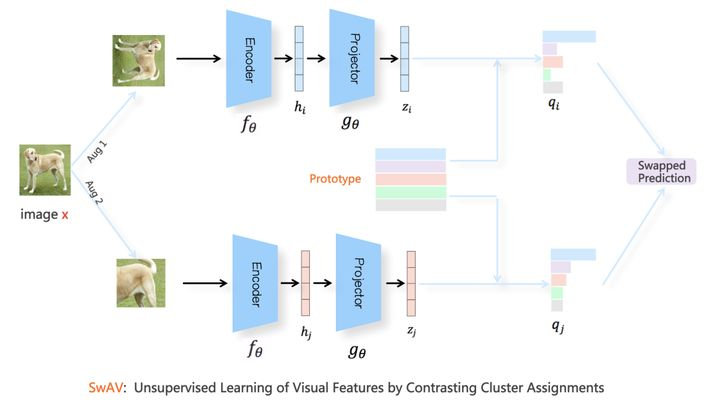SwAV也是上下分枝对称结构，我们以一个例子阐述其工作流程。对于Batch内某张图像 $x$来说，假设其经过图像增强后，在Aug1和Aug2里的对应的增强后图像分别是 $x_i$$x_j$，数据对 $\langle x_i,x_i^+ \rangle$互为正例。增强视图 $x_i$ 走上分枝，经过$g_{\theta}(f_{\theta}(x_i))$ 投影到单位超球面中某点 $z_i$ ，增强视图$x_j$走下分枝，经过$g_{\theta}(f_{\theta}(x_j))$ 投影到单位超球面中某点$z_j$。之后，SwAV对Aug1和Aug2中的表示向量，根据Sinkhorn-Knopp算法，在线对Batch内数据进行聚类。假设走下分枝的$x_j$聚类到了 $q_j$ 类，则SwAV要求表示学习模型根据 $x_i$ 预测 $x_j$ 所在的类，也就是说，要将 $z_i$ 分到第 $q_j$ 类，具体损失函数采用 $z_i$ 和Prototype中每个类中心向量的交叉熵：

$L_{aug1}(z_i,q_j) = -\sum_{k} q_j^k \cdot log(p_i^k)$

$L_{SwAV} = L_{aug1}(z_i,q_j) + L_{aug2}(z_j,q_i)$

SwAV也会面临模型坍塌的问题，具体表现形式为：Batch内所有实例都聚类到同一个类里。所以为了防止模型坍塌，SwAV对聚类增加了约束条件，要求Batch内实例比较均匀地聚类到不同的类别中。

DeepCluster是更早出现的采用两阶段聚类的自监督模型，SwAV论文中对DeepCluser进行了改造，形成了DeepCluser-V2模型。从概念上，可以简单将DeepCluser-V2理解为和SwAV整体结构类似的工作，只不过SwAV对每个Batch数据在线聚类，而DeepCluster-V2是每个Epoch做一次更大规模的聚类。目前来看，SwAV和DeepCluster-V2是效果最好的对比学习模型之一。

### 非对称结构：模型不坍塌之谜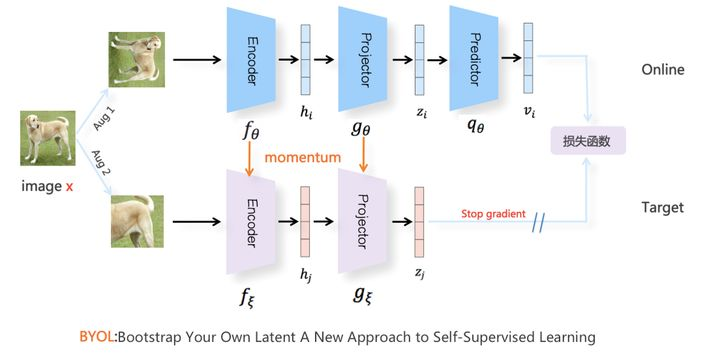BYOL的模型结构如上图所示。对于Batch内任意图像，类似SimCRL采取随机图像增强，产生两组增强图像视图Aug1和Aug2，彼此互为正例，分别走上下两个模型分枝，上分枝被称为Online，下分枝被称为Target。Online分枝的Encoder和Projector和其它对比学习模型是一样的，但是，在Projector之后，新增了一个非线性变换模块Predictor，Predictor的结构和Projector类似（[FC->BN->ReLU->FC]构成的MLP映射网络），产生表示向量 $v_i$并对 $v_i$做L2正则化，将向量映射到单位超球面上。Target分枝结构类似Moco V2对应下分枝的动量更新结构，即由自有参数的Encoder和Projector构成，且模型参数不参与梯度更新，采用Online分枝对应结构参数的Moving Average动量更新方式。以此方式，产生增强图像Aug2的向量 $z_j$，同样地，会对 $z_j$ 做L2正则化操作，将表示向量映射到单位超球面上。但是，因为BYOL不用负例，所以并不需要维护Moco V2中的负例队列，下分枝只是对Aug2中的正例进行投影。

$L_{aug1} = \Vert v_i - v_j \Vert_2^2 = 2-2 \cdot \langle v_i ,z_j \rangle / (\Vert v_i \Vert_2 \cdot \Vert z_j \Vert_2)$

$L_{byol} = L_{aug1}+L_{aug2}$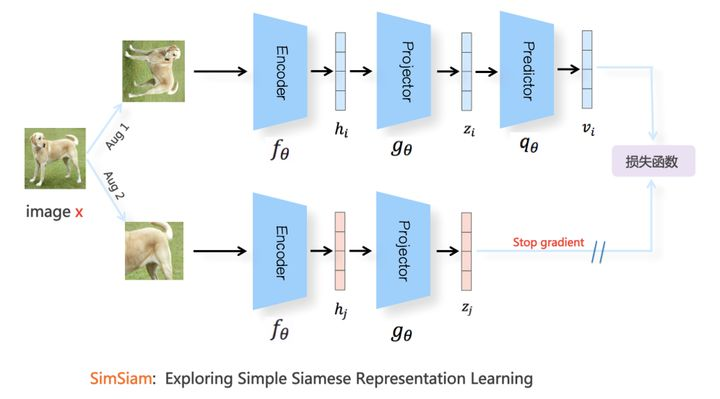SimSiam进一步对BYOL进行了简化，我们可以大致将SimSiam看作是：把BYOL的动量更新机制移除，下分枝的Encoder及Projector和上分枝对应构件参数共享版本的BYOL（参考上图），类似前面介绍BYOL里说的Predictor加大学习率的版本。但是，从后续文献的实验对比来看，SimSiam效果是不及BYOL的，这说明动量更新机制尽管可能不是防止模型坍塌的关键因素，但是对于提升对比学习模型效果是很重要的。

### 冗余消除损失：越简单越快乐$L_{bt} = \sum_i (1 - C_{ii})^2 + \lambda \sum_i \sum_{(j \neq i)} C_{ij}^2$

### 王者或青铜：经典对比学习模型效果比较